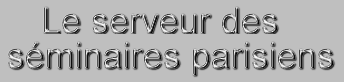Statut Confirmé Série SEM-DARBOUX Domaines hep-th Date Lundi 30 Mars 2015 Heure 16:30 Institut LPTHE Salle bibliothèque du LPTHE Nom de l'orateur Vlassopoulos Prenom de l'orateur Yiannis Addresse email de l'orateur Institution de l'orateur Titre Calabi-Yau algebras and Topological Quantum Field Theories Résumé A Calabi-Yau ($CY$) algebra is a differential graded (or more generally $A_\infty$) algebra with finite dimensional cohomology and a certain kind of duality. An example is the de Rham algebra of forms on a compact closed oriented manifold. Given a $CY$ algebra one can construct a 1+1 dimensional topological quantum field theory (TQFT). $CY$ algebras appear on both sides of the mirror symmetry conjecture for compact $CY$ manifolds, coming from string theory. We shall explain how to generalize $CY$ algebras to the case where the cohomology of the algebra is not necessarily finite dimensional and the duality condition is relaxed. Such algebras are called $pre-CY$ and examples include the de Rham algebra of forms on a manifold with boundary. We shall explain how to construct a 1+1 dimensional TQFT from a $pre-CY$ algebra, via acyclic directed ribbon graphs. $Pre-CY$ algebras appear on both sides of the mirror symmetry conjecture for open $CY$ manifolds and for Fano manifolds. This is joint work with Maxim Kontsevich. Numéro de preprint arXiv Commentaires Fichiers attachés

Pour obtenir l' affiche de ce séminaire : [ Postscript | PDF ]

 [ Annonces ]    [ Abonnements ]    [ Archive ]    [ Aide ]    [ JavaScript requis ] [ English version ]